Instructions

For the following questions answer them individually

Question 1

# A thin and uniform rod of mass M and length L is held vertical on a floor with large friction. The rod is released from rest so that it falls by rotating about its contact-point with the floor without slipping. Which of the following statement(s) is/are correct, when the rod makes an angle $$60^\circ$$ with vertical?[g is the acceleration due to gravity]Question 2

# A block of mass 2M is attached to a massless spring with spring-constant k. This block is connected to two other blocks of masses M and 2M using two massless pulleys and strings. The accelerations of the blocks are $$a_1, a_2$$ and $$a_3$$ as shown in the figure. The system is released from rest with the spring in its unstretched state. The maximum extension of the spring is $$x_0$$. Which of the following option(s) is/are correct?[g is the acceleration due to gravity. Neglect friction]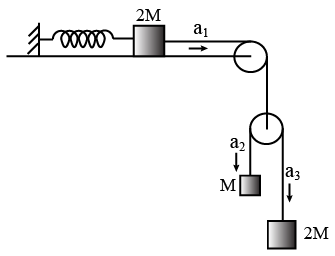Question 3

# A small particle of mass m moving inside a heavy, hollow and straight tube along the tube axis undergoeselastic collision at two ends. The tube has no friction and it is closed at one end by a flat surface while the other endis fitted with a heavy movable flat piston as shown in figure. When the distance of the piston from closed end is $$L = L_0$$ the particle speed is $$v = v_0$$. The piston is moved inward at a very low speed V such that $$V \ll \frac{dL}{L} v_0,$$ where dL is the infinitesimal displacement of the piston. Which of the following statement(s) is/are correct?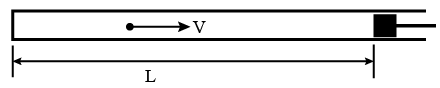Question 4

# An electric dipole with dipole moment $$\frac{p_o}{\sqrt 2} (\widehat{l}\widehat{J})$$ is held fixed at the origin O in the presence of an uniform electric field of magnitude $$E_0$$. If the potential is constant on a circle of radius R centered at the origin as shown in figure, then the correct statement(s) is/are:($$\epsilon_0$$ is permittivity of free space. $$R \gg$$ dipole size)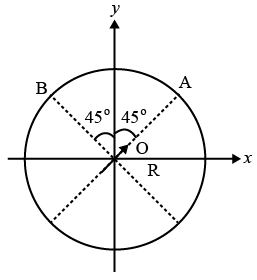Question 5

# A mixture of ideal gas containing 5 moles of monatomic gas and 1 mole of rigid diatomic gas is initially at pressure $$P_0$$, volume $$V_0$$, and temperature $$T_0$$. If the gas mixture is adiabatically compressed to a volume $$\frac{V_0}{4}$$, then the correct statement(s)is/are,(Given $$2^{1.2} = 2.3; 2^{3.2} = 9.2;$$ R is gas constant)Question 6

# Three glass cylinders of equal height H = 30 cm and same refractive index n = 1.5 are placed on a horizontal surface as shown in figure. Cylinder I has a flat top, cylinder II has a convex top and cylinder III has a concave top. The radu of curvature of the two curved tops are same (R = 3 m). If $$H_1, H_2$$ and $$H_3$$ are the apparent depths of a point_X on the bottom of the three cylinders, respectively, the correct statement(s) is/are: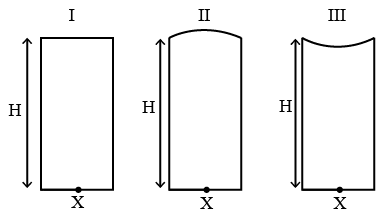Question 7

# In a Young’s double slit experiment, the slit separation d is 0.3 mm and the screen distance D is 1 m. A parallel beam of light of wavelength 600 nm is incident on the slits at angle $$\alpha$$ as shown in figure. On the screen, the point O is equidistant from the slits and distance PO is 11.0 mm. Which of the following statement(s) is/are correct?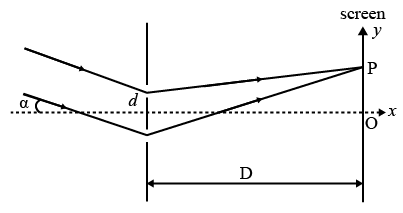Question 8

# A free hydrogen atom after absorbing a photon of wavelength $$\lambda_a$$ gets excited from the state n = 1 to the state n = 4. Immediately after that the electron jumps to n = m state by emitting a photon of wavelength $$\lambda_e$$. Let the change in momentum of atom due to the absorption and the emission are $$\triangle p_a$$ and $$\triangle p_e,$$ respectively. If $$\frac{\lambda_a}{\lambda_e} = \frac{1}{5}$$ which of the option(s) is/are correct?[Use hc = 1242 eV nm ; 1 nm = $$10^{-9}$$ m, h and c are Planck’s constant and speed of light, respectively]Question 9

# A ball is thrown from ground at an angle $$\theta$$ with horizontal and with aninitial speed $$\mu_0$$. For the resulting projectile motion, the magnitude of average velocity of the ball up to the point when it hits the ground for the first time is $$V_1$$. After hitting the ground, the ball rebounds at the same angle $$\theta$$ but with a reduced speed of $$\frac{\mu_0}{\alpha}$$. Its motion continues for a long time as shown in figure. If the magnitude of average velocity of the ball for entire duration of motion is $$0.8 V_1,$$ the value of $$\alpha$$ is __________ .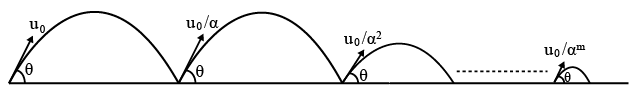Backspace
789
456
123
0.-
Clear AllQuestion 10

# A 10 cm long perfectly conducting wire PQ is moving with a velocity 1 cm/s on a pair of horizontal rails of zero resistance. One side of the rails is connected to an inductor L = 1 mH and a resistance $$R = 1 Ω$$ as shown in figure. The horizontal rails, L and R lie in the same plane with a uniform magnetic field B = 1 T perpendicularto the plane. If the key S is closed at certain instant, the current in the circuit after 1 millisecond is $$x \times 10^{-3} A$$, where the value of x is ___________ .[Assume the velocity of wire PQ remains constant (1 cm/s) after key S is closed. Given: $$e^{-1} = 0.37$$, where e is base of the natural logarithm ]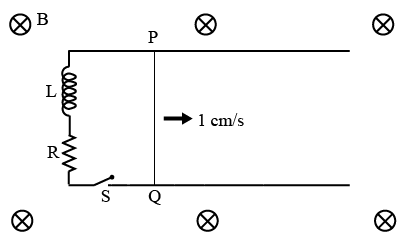Backspace
789
456
123
0.-
Clear AllOR